•文档
• 对于一个矩阵初等变换，有三种： 1. 交换两行。 2. 将某一行的所有元素乘以一个非零实数kk。 3. 将某一行jj，加上某一行i(i≠ji(i \not = j)乘以一个非零实数kk，即Aj=Aj+Ai∗kA_j = A_j + A_i * k。可以发现...
设要求出

n
$n$阶矩阵A$A$的逆矩阵

B
$B$。

对于一个矩阵的初等行变换，有三种：
1. 交换两行。
2. 将某一行的所有元素乘以一个非零实数k$k$。  3. 将某一行

j
$j$，加上某一行i(i≠j$i(i \not = j$)乘以一个非零实数

k
$k$，即Aj=Aj+Ai∗k$A_j = A_j + A_i * k$。
可以发现的是，每种变换其实都可以等价于乘以某个矩阵，事实上称其为初等矩阵。
那么，当我们不停地对

A
$A$进行初等变换，并且用另外一个矩阵C$C$不停地乘上这种变换对应的初等矩阵，那么当

A
$A$变为I(单位矩阵)$I(单位矩阵)$时，

C
$C$就是A$A$的逆矩阵了。  怎么样将

A
$A$变为I$I$？我们类似于高斯消元一样，一行一行一列一列地扫过去。由于最终要保证

Ai,i=1
$A_{i,i} = 1$，其他为

0
$0$。
设当前扫到第i$i$行，那么对于

Ai,1∼i−1=0
$A_{i,1\sim i - 1} = 0$。但是对于

j<i，Aj,i
$j < i，A_{j,i}$可能不等于0。但我们初等变换中可以先对第

i
$i$行除以Ai,i$A_{i,i}$，即保证

Ai,i=1
$A_{i,i} = 1$，接着用

i
$i$整行去消j<i$j < i$。那么

Aj,i
$A_{j,i}$就等于0了。那么我们这样一行一行地消下去即可。我们对

A
$A$中做的所有操作，顺便对C$C$同时做就好了。反正都是乘上同一个矩阵。
一开始没有操作时

C
$C$就是I$I$。
最后我们用

O(N3)
$O(N^3)$的复杂度求出了逆矩阵。
展开全文• 复数矩阵求逆，用了STL中的vector表示矩阵，用了complex类表示复数，求矩阵使用了初等变换矩阵的方法
• python def compute_inverse(A): row, col = A.shape ...从线代角度来看感觉没什么问题，但是测试的时候发现对4阶以下的矩阵能够正确求得其逆矩阵，但是高于5阶就只能偶尔正确，不知道出现了什么问题python python
• 下面是通过初等变换求解逆矩阵的，非用伴随矩阵! 比如： 1 2 3 2 2 1 3 4 3 则把下面矩阵： 1 2 3 1 0 0 2 2 1 0 1 0 3 4 3 0 0 1 通过初等变换变为： 1 0 0 x x x 0 1 0 x x x 0 0 1 x x x 则x矩阵就是要求的...
• 1. 将矩阵A变换为单位矩阵的同时，经过同等变换的单位矩阵将变换为矩阵A的逆矩阵 2. 逆矩阵的计算示例 （行变换） 3.逆矩阵的计算示例 （列变换） 4. 利用初等变换求逆矩阵 ...
1. 将矩阵A变换为单位矩阵的同时，经过同等变换的单位矩阵将变换为矩阵A的逆矩阵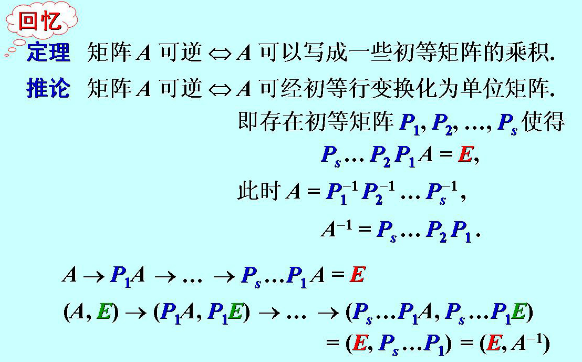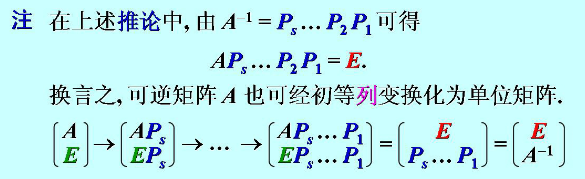2. 逆矩阵的计算示例 （行变换）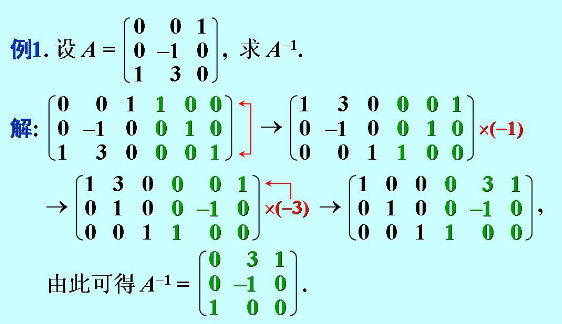3. 逆矩阵的计算示例 （列变换）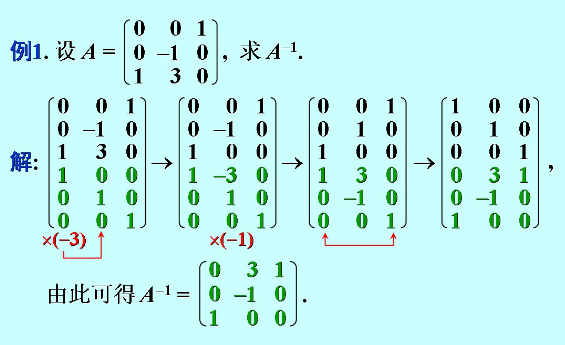4. 利用初等变换求逆矩阵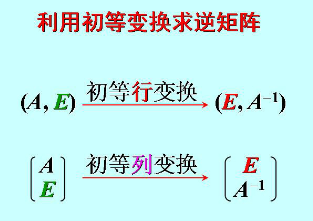展开全文• 在我们学习逆矩阵的过程中，肯定会遇到这样一种求逆矩阵的方法：若A是一个n阶可逆矩阵， （虚竖线打不出，凑合着看吧！）这一过程中经历了一系列初等变换，并且我们下结论说： 中的 就是A的逆矩阵！按逻辑，你还不...在我们学习逆矩阵的过程中，肯定会遇到这样一种求逆矩阵的方法：
若A是一个n阶可逆矩阵，（虚竖线打不出，凑合着看吧！）这一过程中经历了一系列初等行变换，并且我们下结论说：中的就是A的逆矩阵！

按逻辑，你还不能确定这个就是它的逆矩阵，你现在还没有理由确定，所以我们暂定这个=B

我的意思就是将一系列的初等行变换补到表达中去的话，将会是如下这个样子：即则对左边左半部分 有又对左边右半部分有也即则此时，我们才有理由说中的就是A的逆矩阵！
这里就是我们的行变换！
那我们要进行列变换该怎么办，只要右乘矩阵就可以。
而为什么可以这样，就还得了解矩阵的线性组合了。（这以后我再试着说一说吧，我们老师还没讲到那里来，也有一些逻辑关系要弄清。有兴趣的话，私下我们一起交流啊！！）
例题：,用初等变换求A的逆矩阵。

解：这一题还有个很神奇很巧合的解法
法二：真的是太巧合了。

开开心心地开始学线代！

展开全文ker矩阵是什么意思
• c#好像没有自带的矩阵类，而很多的算法中需要用到矩阵类，今天分享一个c#实现的矩阵类，包含了矩阵的加，乘法，转置求（伴随矩阵求逆：当行列式的值特别大或特别小的时候不适用。初等变换：求效率要高，不受行列...
c#好像没有自带的矩阵类，而很多的算法中需要用到矩阵类，今天分享一个c#实现的矩阵类，包含了矩阵的加，乘法，转置求逆（伴随矩阵求逆：当行列式的值特别大或特别小的时候不适用。初等变换：求逆效率要高，不受行列式值的影响)。求行列式的值等功能具体代码如下：
 /// <summary>
/// 矩阵类
/// </summary>
public class Mat
{
double[,] Array;//存储矩阵中的元素
public double[,] Element => Array;//获取矩阵中的元素
public int Rows => Element.GetLength(0);//行
public int Cols => Element.GetLength(1);//列
public double this[int i,int j]//索引器
{
get
{
return Array[i, j];
}
set
{
Array[i, j] = value;
}
}
/// <summary>
/// 构造函数
/// </summary>
/// <param name="r">矩阵的行</param>
/// <param name="c">矩阵的列</param>
/// <param name="n">矩阵的元素</param>
public Mat(int r,int c,params double [] n)
{
Array = new double[r, c];
for (int i = 0; i < Rows; i++)
{
for (int j = 0; j < Cols; j++)
{
Array[i, j] = n[i * Cols + j];
}
}
}
/// <summary>
/// 构造r行n列的空矩阵
/// </summary>
/// <param name="r">行</param>
/// <param name="c">列</param>
public Mat(int r,int c)
{
Array = new double[r, c];
}
/// <summary>
/// 将一个二位数组构造为矩阵
/// </summary>
/// <param name="array"></param>
public Mat(double [,] array)
{
this.Array = array;
}
/// <summary>
/// 将n个行列相同的矩阵向下累加
/// </summary>
/// <param name="mats"></param>
public Mat(params Mat []mats)
{
this.Array = new double[mats.Length * mats.Rows, mats.Cols];
for (int n = 0; n < mats.Length; n++)
{
for (int i = 0; i < mats.Rows; i++)
{
for (int j = 0; j < mats.Cols; j++)
{
Array[n * mats.Rows + i, j] = mats[n][i, j];
}
}
}
}
/// <summary>
/// 矩阵的加法
/// </summary>
/// <param name="m1">左矩阵</param>
/// <param name="m2">右矩阵</param>
/// <returns></returns>
public static Mat operator +(Mat m1, Mat m2)
{
Mat res = new Mat(m1.Rows, m1.Cols);
for (int i = 0; i < m1.Rows; i++)
{
for (int j = 0; j < m1.Cols; j++)
{
res[i, j] = m1[i, j] + m2[i, j];
}
}
return res;
}
/// <summary>
/// 矩阵的乘法
/// </summary>
/// <param name="m1">左矩阵</param>
/// <param name="m2">右矩阵</param>
/// <returns></returns>
public static Mat operator *(Mat m1, Mat m2)
{
Mat res = new Mat(m1.Rows, m2.Cols);
for (int i = 0; i < m1.Rows; i++)
{
for (int k = 0; k < m2.Cols; k++)
{
double sum = 0;
for (int j = 0; j < m1.Cols; j++)
{
sum += m1[i, j] * m2[j, k];
}
res[i, k] = sum;
}

}
return res;
}
/// <summary>
/// 矩阵于数相乘
/// </summary>
/// <param name="n"></param>
/// <returns></returns>
public Mat Mul(double n)
{
Mat res = new Mat(Rows, Cols);
for (int i = 0; i < Rows; i++)
{
for (int j = 0; j < Cols; j++)
{
res[i, j] = n * Array[i, j];
}
}
return res;
}
/// <summary>
/// 行列式的值
/// </summary>
public double MatValue
{
get
{
if (Rows == 1)
{
return Array[0,0];
}
else
{
double sum = 0;
for (int i = 0; i < Cols; i++)
{
sum += Array[0,i] * GetK(0, i) * Accompany(0, i).MatValue;
}
return sum;
}
}
}
/// <summary>
/// 伴随矩阵求逆
/// </summary>
public Mat Inverser
{
get
{
double value = MatValue;
Mat matrix = new Mat(Rows, Cols);
for (int i = 0; i < Rows; i++)
{
for (int j = 0; j < Cols; j++)
{
matrix.Array[i, j] = (GetK(j, i) * Accompany(j, i).MatValue / value);
}
}
return matrix;
}
}
/// <summary>
/// 矩阵的转置
/// </summary>
public Mat TransMat
{
get
{

Mat res = new Mat(Cols, Rows);
for (int i = 0; i < Rows; i++)
{
for (int j = 0; j < Cols; j++)
{
res[j, i] = this[i, j];
}
}
return res;
}
}
/// <summary>
/// 矩阵第r行第c列的的伴随矩阵
/// </summary>
/// <param name="r"></param>
/// <param name="c"></param>
/// <returns></returns>
public  Mat Accompany(int r, int c)
{
Mat res = new Mat(Rows - 1, Cols - 1);
for (int i = 0; i < res.Rows; i++)
{
for (int j = 0; j < res.Cols; j++)
{
res[i, j] = this[i < r ? i : i + 1, j < c ? j : j + 1];
}
}
return res;
}

/// <summary>
/// 初等变换求逆
/// </summary>
public Mat Inverser2
{
get
{

int  m = Rows;
int   n = Cols;
double[,] array = new double[2 * m + 1, 2 * n + 1];
for (int k = 0; k < 2 * m + 1; k++)  //初始化数组
{
for (int t = 0; t < 2 * n + 1; t++)
{
array[k, t] = 0.00000000;
}
}
for (int i = 0; i < m; i++)
{
for (int j = 0; j < n; j++)
{
array[i, j] = Array[i, j];
}
}

for (int k = 0; k < m; k++)
{
for (int t = n; t <= 2 * n; t++)
{
if ((t - k) == m)
{
array[k, t] = 1.0;
}
else
{
array[k, t] = 0;
}
}
}
//得到逆矩阵
for (int k = 0; k < m; k++)
{
if (array[k, k] != 1)
{
double bs = array[k, k];
array[k, k] = 1;
for (int p = k + 1; p < 2 * n; p++)
{
array[k, p] /= bs;
}
}
for (int q = 0; q < m; q++)
{
if (q != k)
{
double bs = array[q, k];
for (int p = 0; p < 2 * n; p++)
{
array[q, p] -= bs * array[k, p];
}
}
else
{
continue;
}
}
}
double[,] NI = new double[m, n];
for (int x = 0; x < m; x++)
{
for (int y = n; y < 2 * n; y++)
{
NI[x, y - n] = array[x, y];
}
}
return new Mat(NI);
}
}
private int GetK(int r,int c)
{
return (int)Math.Pow(-1, r + c);
}
/// <summary>
/// 矩阵的ToString方法
/// </summary>
/// <returns></returns>
public override  string ToString()
{
string str = "";
for (int i = 0; i < Rows; i++)
{
for (int j = 0; j < Cols; j++)
{
}
str += "\r\n" + "\r\n";
}
return str;
}
}

展开全文c#
• 初等变换法求解λ矩阵的不变因子 当然也可以用行列式因子求解
•c语言 C++
• 逆矩阵求法行列式初等变换 在前面我们以学习了用公式求逆矩阵，但当矩阵A的阶数较大时，求A*很繁琐，此方法不实用，因此必须找一种更简单的方法求逆矩阵，那么如何找到一种简单的方法呢？
• 实数矩阵求逆，用了STL中的vector表示矩阵，求矩阵使用了初等变换矩阵的方法
•线性代数 行列式
• c++ 编写的逆矩阵求法的代码   #include #include using namespace std; static double a; static double b; void the_data_change_to_1(double a[],double b[],int m,int n);//...
• O(n5)O(n^5)O(n5) 做法： 先出 AAA 的伴随矩阵 A∗...首先介绍矩阵初等变换（以下为初等行变换）： 交换两行，记做 ri:left-right_arrow:rjr_i\leftrightarrow r_jri​:left-right_arrow:rj​ 将一行的所有元乘上数
•线性代数 行列式
• P4783 【模板】矩阵求逆 题目描述 求一个N×NN×NN×N的矩阵的矩阵。答案对109+710^9+7109+7取模。 1.矩阵的定义 假设 AAA 是一个方阵，如果存在一个矩阵 A−1A^{-1}A−1，使得 A−1A=IA^{-1}A=IA−1A=I 并且 AA...
• 线性方程组是线性代数学课的考虑范畴，会使用matlab解决，可以效率更快。matlab种逆矩阵是左除和右除。初等变换法就是一个rref函数matlab 数学建模
• 初等变换求矩阵的秩初等变换求矩阵的秩初等变换求矩阵的秩初等变换求矩阵的秩初等变换求矩阵的秩
• 高等代数的理论知识(A | E) 经过初等变换（E | A-1）（A-1 代表A的）matrix_inv &lt;- function(A) { A_zhi &lt;- Matrix::rankMatrix(A) n_row &lt;- dim(A) n_col &lt;- dim(A) ...
• 一、初等变换 二、初等矩阵 2.1、初等矩阵定义
• 本篇笔记首先回顾了伴随矩阵法求逆矩阵，因为过程过于复杂，所以引出初等变换法求逆矩阵，并推导了初等变换法求逆矩阵的思路；然后通过一个例子介绍了初等变换法求逆矩阵的过程，并对注意事项进行了总结；最后还讨论...线性代数...